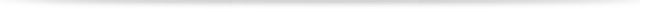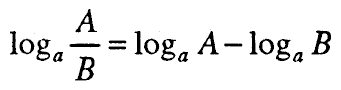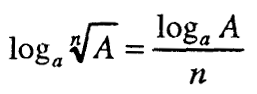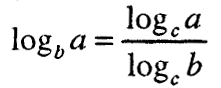# Logarithm

## 1. NOTION OF ACCOUNT

A logarithm for a given positive number b for a given basis a, a> 0 and a ≠ 1, is an indicator c with which the base a should be graded a to obtain the number b, ie.Thereby, b is called a numerus or logarithm, a – base of the logarithm and c – logarithm of b for base a.

The definition of a logarithm follows the equation:which is called basic identity in logarithm theory.

The following properties (a> 0 and a ≠ 1) follow directly from the definition of a logarithm:

1 °. logₐ1 = 0, since а⁰ = 1

2 °. logₐa = 1, because а¹ = а

3. logͣaⁿ = n, because aⁿ = aⁿ## 2. RULES FOR ACCOUNTING

### 2.1. Product logarithm

The logarithm of the product of the positive numbers A and B for a given basis a (a> 0 and a ≠ 1) is equal to the sum of the logarithms of the multipliers for the same basis, ie.

logₐAB = logₐA + logₐB

### 2.2. Quantitative logarithm

The logarithm of the quotient of the positive numbers A and B for a given basis a (a> 0 and a ≠ 1) is equal to the difference between the logarithm of the numerator and the logarithm of the denominator for the same basis, ie.### 2.3. Degree logarithm

Logarithm of degree with positive base a, a а 1, equal to the product of the indicator of that degree and the logarithm of its basis, ie.

logₐAⁿ = nlogₐA

### 2.4. Root logarithm

A logarithm of a root of a positive number is equal to the quotient of the logarithm of the root size and the index of the root, ie.## 3. RELATIONSHIP BETWEEN ACCOUNTINGS WITH DIFFERENT BASIS

Let a, b and c be real positive numbers and let b and c. 1.

The formulagives the relation between the logarithms of the number a on different bases b and c.## 4. CALCULATION AND ANTILOGARITIMATION OF EXPRESSIONS

Logarithm of an algebraic expression is performed based on the rules for logarithm of product, quotient, degree and root and the properties of the logarithms.

Finding an algebraic expression whose logarithm is known is called antilogarithmization.

– logarithmization –

Total Page Visits: 237 - Today Page Visits: 1